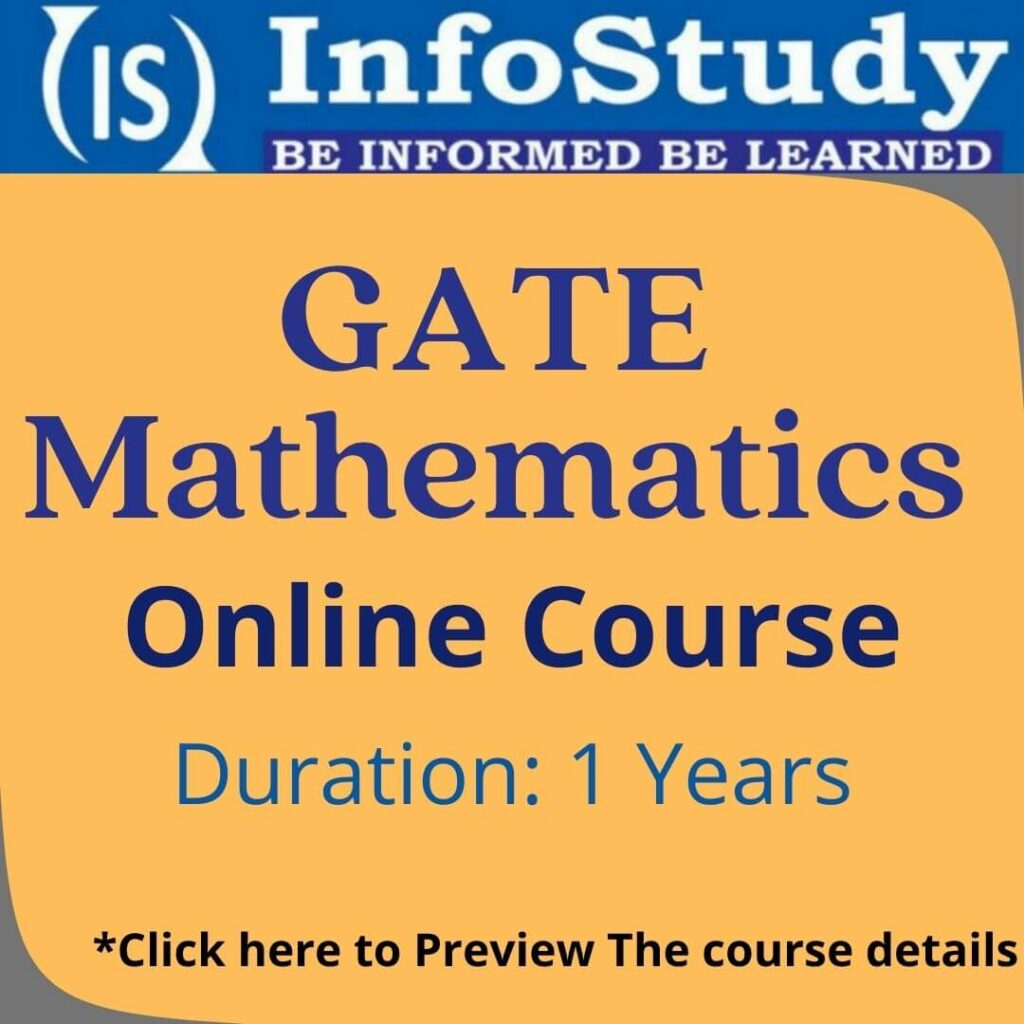# GATE Mathematics

GATE Stands For Graduate Aptitude Test in Engineering. GATE is a national level entrance exam conducted for Engineering Students by the Indian Institute of Sciences (IISc) & the 7 Institute of Technology (IITs) at Bombay, Delhi, Guwahati, Kanpur, Kharagpur, Madras, and Roorkee. A GATE score is used for M.Tech, Ph.D. admission, and various PSU Recruitment & Jobs. GATE Mathematics is very scoring for those who have a strong mathematics background.

This course has been framed specially for the aspirants of Gate Mathematics,  and it will contribute significantly towards the attainment of a good rank in Gate MA Exam. The course at GATE mathematics coaching in Delhi consists of video lectures, doubt session, various online and offline tests and PREVIOUS PAPER DISCUSSION on the following topics: Complex Analysis, Ordinary Differential Equations, Partial Differential Equations, Linear Algebra, Modern Algebra, Real Analysis, Topology, Numerical Analysis, and Linear Programming Problems (LPP). This course will not only be beneficial for students appearing for GATE Mathematics, but also for aspirants of NTA CSIR UGC NET MATHS, TIFR and NBHM. Confidence to face an interview will be an extra advantage. If you watch all the videos and do all the assignments of this course sincerely.

## Eligibility Criteria for GATE Exam

• Qualifying Degree – B.E /B.Tech
• Applicant Type – An applicant who had completed B.E or B.Tech in Engg and those who are in the final year of such programs with no backlog.
• There is No Age limit.

## Examination Centers For GATE

• IISc Bangalore
• IIT Bombay
• IIT Delhi, Hauz Khas
• IIT Guwahati
• IIT Kanpur

## The pattern of GATE Mathematics

This paper will be for 3 hours duration and there will be a total of 65 questions carrying 100 marks, out of which 10 questions carrying a total of 15 marks will be on General Aptitude (GA). In the paper, the General Aptitude section will carry 15% of the total marks, and the remaining 85% of the total marks are devoted to the subject of the paper. This GATE MA paper would contain questions of two different types:

(i) Multiple Choice Questions (MCQ) carrying 1 or 2 marks each in all sections. These questions are objective in nature and each will have a choice of four answers, out of which the candidate has to mark the correct answer(s).

(ii) Numerical Answer Type Questions (NAT) carrying 1 or 2 marks each in all sections. For these questions the answer is a real number, to be entered by the candidate using the virtual keypad on the monitor (the keyboard of the computer will be disabled). No choices will be shown for this type of question. The answer can be a number such as 10 (an integer only). The answer may be in decimals as well, for example, 10.1 (one decimal) or 10.01 (two decimals), or 10.001 (three decimals). These questions will be mentioned with, up to which decimal places, the candidates need to present the answer. Also, for some NAT type problems an appropriate range will be considered while evaluating the numerical answer type questions so that the candidate is not unduly penalized due to the usual round-off errors. Wherever required and possible, it is better to give NAT answers up to a maximum of three decimal places.

## Marking Scheme

For 1-mark multiple-choice questions, 1/3 mark will be deducted for a wrong answer. Likewise, for 2-mark multiple-choice questions, 2/3 marks will be deducted for a wrong answer. There is NO negative marking for numerical answer-type questions.

## General Aptitude (GA) Questions

In the paper, GA questions carry a total of 15 marks. The GA section includes 5 questions carrying 1-mark each (sub-total 5 marks) and 5 questions carrying 2-marks each (sub-total 10 marks).## Subject Questions

The paper would contain 25 questions carrying 1-mark each (subtotal 25 marks) and 30 questions carrying 2-marks each (sub-total 60 marks). All in all, Infostudy, GATE mathematics coaching in Delhi, helps each student in the preparations for exams.